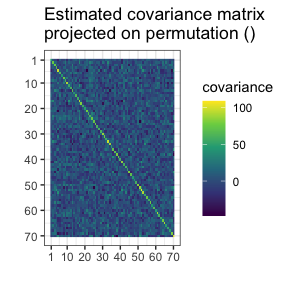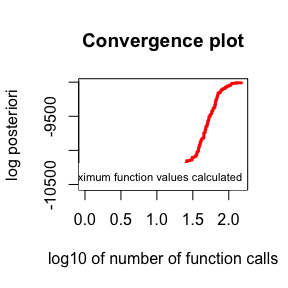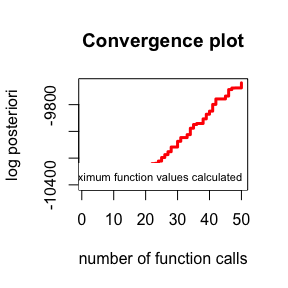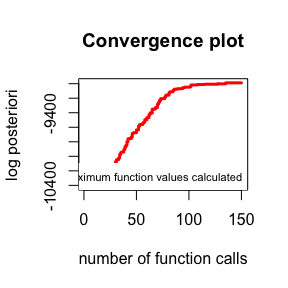# Optimizers

library(gips)
?find_MAP()

# What are we optimizing?

The goal of the find_MAP() is to find the permutation $$\sigma$$ that maximizes the a posteriori probability (MAP - Maximum A Posteriori). Such a permutation represents the most plausible symmetry given the data.

This a posteriori probability function is described in-depth in the Bayesian model selection section of the vignette("Theory", package="gips"), also available as a pkgdown page. gips can calculate the logarithm of it by the log_posteriori_of_gips() function. In the following paragraphs, we will refer to this a posteriori probability function as $$f(\sigma)$$. We have $$f(\sigma) > 0$$.

# Available optimizers

The space of permutations is enormous - for the permutation of size $$p$$, the space of all permutations is of size $$p!$$ ($$p$$ factorial). Even for $$p=12$$, this space is practically impossible to browse. This is why find_MAP() implements multiple (3) optimizers to choose from:

• "brute_force", "BF", "full" | recommend for $$p\le 9$$.
• "Metropolis_Hastings", "MH" | recommend for $$p\ge 10$$.
• "hill_climbing", "HC"

### Note on computation time

The max_iter parameter functions differently in Metropolis-Hastings and hill climbing.

For Metropolis-Hastings, it computes a posteriori of max_iter permutations, whereas for hill climbing, it computes $${p\choose 2} \cdot$$ max_iter of them.

In the case of the Brute Force optimizer, it computes all $$f(\sigma)$$ values. The number of all different $$\sigma$$s follows OEIS sequence A051625.

## Brute Force

It searches through the whole space at once.

This is the only optimizer that will certainly find the actual MAP Estimator.

Brute Force is only recommended for small spaces ($$p \le 9$$). It can also browse bigger spaces, but the required time is probably too long. We tested how much time it takes to browse with Brute Force (AMD EPYC 7413 Processor, single core), and we show the time in the table below:

p=2 p=3 p=4 p=5 p=6 p=7 p=8 p=9 p=10
0.007 sec 0.015 sec 0.04 sec 0.2 sec 1 sec 4.5 sec 30 sec 4 min 1 hour 45 min

### Example

Let’s say we have the data Z from the unknown process:

dim(Z)
#>  13  6
number_of_observations <- nrow(Z) # 13
perm_size <- ncol(Z) # 6
S <- cov(Z) # Assume we have to estimate the mean

g <- gips(S, number_of_observations)

g_map <- find_MAP(g, optimizer = "brute_force")
#> ================================================================================
g_map
#> The permutation (1,2,3,4,5,6):
#>  - was found after 362 posteriori calculations;
#>  - is 28979.967 times more likely than the () permutation.

Brute Force needed 362 calculations, as predicted in OEIS sequence A051625 for $$p = 6$$.

## Metropolis-Hastings

This optimizer implements the Second approach from [1, Sec 4.1.2].

It uses the Metropolis-Hastings algorithm to optimize the space; see Wikipedia. This algorithm used in this context is a special case of the Simulated Annealing the reader may be more familiar with; see Wikipedia.

### Short description

In every iteration $$i$$, an algorithm considers a permutation, say, $$\sigma_i$$. Then a random transposition is drawn uniformly $$t_i = (j,k)$$, and the value of $$f(\sigma_i \circ t_i)$$ is computed.

• If a new value is bigger than the previous one (i.e., $$f(\sigma_i \circ t_i) \ge f(\sigma_i)$$), then we set $$\sigma_{i+1} = \sigma_i \circ t_i$$.
• If a new value is smaller ($$f(\sigma_i \circ t_i) < f(\sigma_i)$$), then we will choose $$\sigma_{i+1} = \sigma_i \circ t_i$$ with probability $$\frac{f(\sigma_i \circ t_i)}{f(\sigma_i)}$$. Otherwise, we set $$\sigma_{i+1} = \sigma_i$$ with complementary probability $$1 - \frac{f(\sigma_i \circ t_i)}{f(\sigma_i)}$$.

The final value is the best $$\sigma$$ ever computed.

### Example

Let’s say we have the data Z from the unknown process:

dim(Z)
#>  50 70
number_of_observations <- nrow(Z) # 50
perm_size <- ncol(Z) # 70
S <- cov(Z) # Assume we have to estimate the mean

g <- gips(S, number_of_observations)
suppressMessages( # message from ggplot2
plot(g, type = "heatmap") +
ggplot2::scale_x_continuous(breaks = c(1, 10, 20, 30, 40, 50, 60, 70)) +
ggplot2::scale_y_reverse(breaks = c(1, 10, 20, 30, 40, 50, 60, 70))
)g_map <- find_MAP(g, max_iter = 150, optimizer = "Metropolis_Hastings")
#> ===============================================================================
g_map
#> The permutation (1,59,52,69,20,47,5,3,65,66,16,44,56,26,60,42,28,15,10,29,41,53,61,19,9,48,70,12,17,14,32,43,13,45,23,62,36,24,68,39,38,34,33,35,50,46,30,4,57,31,8,54,67,40,37,21,63,64,7,49,58,6,27,55,18,11,25,51):
#>  - was found after 150 posteriori calculations;
#>  - is 3.904e+656 times more likely than the () permutation.

After just a hundred and fifty iterations, the found permutation is unimaginably more likely than the $_0 =$ () permutation.

plot(g_map, type = "best", logarithmic_x = TRUE)# Continuing the optimization

When max_iter is reached during Metropolis-Hastings or hill climbing, the optimization stops and returns the result. Users are encouraged to plot the result and determine if it has converged. If necessary, users can continue the optimization, as shown below.

g <- gips(S, number_of_observations)

g_map <- find_MAP(g, max_iter = 50, optimizer = "Metropolis_Hastings")
#> ==============================================================================
plot(g_map, type = "best")The algorithm was still significantly improving the permutation. It is reasonable to continue it:

g_map2 <- find_MAP(g_map, max_iter = 100, optimizer = "continue")
#> ===============================================================================
plot(g_map2, type = "best")The improvement has slowed down significantly. It is fair to stop the algorithm here. Keep in mind the y scale is logarithmic. The visually “small” improvement between 100 and 150 iterations was huge, $$10^{52}$$ times the posteriori.

The find_MAP() function has two additional parameters: show_progress_bar and save_all_perms, which can be set to TRUE or FALSE.
When show_progress_bar = TRUE, gips will print “=” characters on the console during optimization. Remember that when the user sets the return_probabilities = TRUE, a second progress bar will indicate the calculation of the probabilities after optimization.
The save_all_perms = TRUE will save all visited permutations in the outputted object, which significantly increases the required RAM. For instance, with $$p=150$$ and max_perm = 150000, we needed 400 MB to store it, whereas save_all_perms = FALSE only required 2 MB. However, save_all_perms = TRUE is necessary for return_probabilities = TRUE or more complex path analysis.